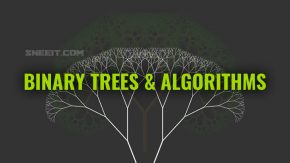# Dynamic Progamming

This course provides you with a framework for solving Dynamic Programming (DP) problems, learn about common patterns, and walk through manyDynamic Programming (DP) is a very important approach in solving problems that can be reduced to smaller problems. For example, you need to find the total ways to climb to the top of a stair which is actually the sum of total ways to climb to the (top – 1)th steps and (top-2)th steps due to the climbing rules that you can take 1 or 2 step at a time.

DP is usually used to find best cases, ex: max/min/largest/smallest/longest/shortest … or counting the total distinct ways to do something

In this course, we will learn every basic aspect of Dynamic Programming follow the below structure:

• Introduction
• What is Dynamic Programming?
• How to Implement DP?
• When to Use DP?
• Strategies
• Framework
• Multidimensional DP
• Time and Space Complexity
• Convert Top-Down to Bottom-Up
• Common Patterns in DP
• Iteration in the Recurrence Relation
• State Transition by Inaction
• State Reduction
• Counting DP
• DP for Path in Matrix

** You will need a solid understanding of recursion, a basic understanding of what greedy algorithms are, and general knowledge such as big O and hash tables.

Start to learn the lessons